# Product rule for partial differentiation

## Statement for two functions

### Statement for partial derivatives

Version type Statement for functions of two variables
specific point, named functions Suppose$f,g$ are both functions of variables$x,y$. Suppose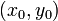$(x_0,y_0)$ is a point in the domain of both$f$ and$g$. Suppose the partial derivatives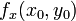$f_x(x_0,y_0)$ and$g_x(x_0,y_0)$ both exist. Let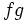$fg$ denote the product of the functions. Then, we have: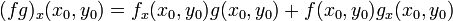$(fg)_x(x_0,y_0) =f_x(x_0,y_0)g(x_0,y_0) + f(x_0,y_0)g_x(x_0,y_0)$
Suppose the partial derivatives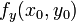$f_y(x_0,y_0)$ and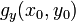$g_y(x_0,y_0)$ both exist. Then, we have:$(fg)_y(x_0,y_0) = f_y(x_0,y_0)g(x_0,y_0) + f(x_0,y_0)g_y(x_0,y_0)$
generic point, named functions Suppose$f,g$ are both functions of variables$x,y$.$(fg)_x(x,y) =f_x(x,y)g(x,y) + f(x,y)g_x(x,y)$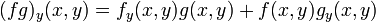$(fg)_y(x,y) = f_y(x,y)g(x,y) + f(x,y)g_y(x,y)$
These hold wherever the right side expressions make sense (see concept of equality conditional to existence of one side).
generic point, named functions, point-free notation Suppose$f,g$ are both functions of variables$x,y$.$(f g)_x =f_xg + fg_x$$(f g)_y = f_yg + fg_y$
These hold wherever the right side expressions make sense (see concept of equality conditional to existence of one side).

### Statement for directional derivatives

Version type Statement
specific point, named functions Suppose$f,g$ are both real-valued functions of a vector variable$\overline{x}$. Suppose$\overline{u}$ is a unit vector. Suppose$\overline{x_0}$ is a point in the domain of both functions. Then, we have the following product rule for directional derivatives:$\! \nabla_{\overline{u}}(fg)(\overline{x_0}) = \nabla_{\overline{u}}(f)(\overline{x_0})g(\overline{x_0}) + f(\overline{x_0})\nabla_{\overline{u}}(g)(\overline{x_0})$
generic point, named functions Suppose$f,g$ are both real-valued functions of a vector variable$\overline{x}$. Suppose$\overline{u}$ is a unit vector. Then, we have the following product rule for directional derivatives wherever the right side expression makes sense (see concept of equality conditional to existence of one side):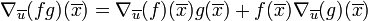$\! \nabla_{\overline{u}}(fg)(\overline{x}) = \nabla_{\overline{u}}(f)(\overline{x})g(\overline{x}) + f(\overline{x})\nabla_{\overline{u}}(g)(\overline{x})$.
generic point, named functions, point-free notation Suppose$f,g$ are both real-valued functions of a vector variable$\overline{x}$. Suppose$\overline{u}$ is a unit vector. Then, we have the following product rule for directional derivatives wherever the right side expression makes sense (see concept of equality conditional to existence of one side):$\! \nabla_{\overline{u}}(fg) = \nabla_{\overline{u}}(f)g + f\nabla_{\overline{u}}(g)$.

The rule applies at all points where the right side make sense.

Version type Statement
specific point, named functions Suppose$f,g$ are both real-valued functions of a vector variable$\overline{x}$. Suppose$\overline{x_0}$ is a point in the domain of both functions. Then, we have the following product rule for gradient vectors:$\! \nabla(fg)(\overline{x_0}) = g(\overline{x_0}) \nabla (f)(\overline{x_0}) + f(\overline{x_0})\nabla (g)(\overline{x_0})$. Note that the products on the right side are scalar-vector multiplications.
generic point, named functions Suppose$f,g$ are both real-valued functions of a vector variable$\overline{x}$. Then, we have the following product rule for gradient vectors wherever the right side expression makes sense (see concept of equality conditional to existence of one side):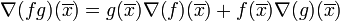$\! \nabla(fg)(\overline{x})= g(\overline{x}) \nabla (f)(\overline{x}) + f(\overline{x})\nabla (g)(\overline{x})$. Note that the products on the right side are scalar-vector function multiplications.
generic point, named functions, point-free notation Suppose$f,g$ are both real-valued functions of a vector variable$\overline{x}$. Then, we have the following product rule for gradient vectors wherever the right side expression makes sense (see concept of equality conditional to existence of one side):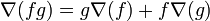$\! \nabla(fg) = g\nabla (f) + f\nabla (g)$. Note that the products on the right side are scalar-vector function multiplications.

## Statement for multiple functions

### Statement for partial derivatives

Fill this in later

### Statement for directional derivatives

Fill this in later